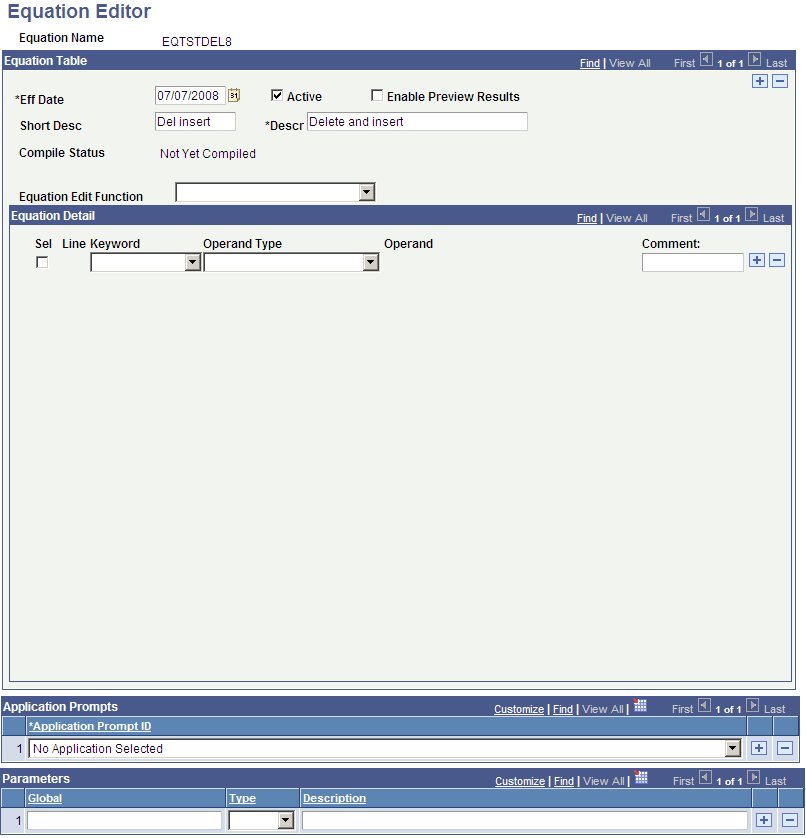Naming Equations

This sections discusses how to name equations.

When you write a new equation, you must give it a name that follows strict naming guidelines. Your IT department will help you establish rules for equations within the institution. Do not use a naming convention that begins with the letters of a PeopleSoft product name or subproduct name. For example, do not use AA (academic advisement), AD (admissions), CC (campus community), CS (campus solutions), FA (financial aid), HR (human resources), SF (student financials), or SR (student records). Each department in the institution should have its own equation name prefix as well to avoid naming conflicts.

Note: You must not modify any system-specific equations because compromising those equations will cause negative processing ramifications for the specific feature.

Page Name

Definition Name

Usage

Equation Editor

EQUATION_EDITOR

select Set Up SACR, then select Common Definitions, then select Equation Engine, then select Equation Editor

Name an equation.

Access the Equation Editor page (select Set Up SACR, then select Common Definitions, then select Equation Engine, then select Equation Editor).

Image: Equation Editor (EQTSTDEL8) page

This example illustrates the fields and controls on the Equation Editor (EQTSTDEL8) page. You can find definitions for the fields and controls later on this page.Create an equation name based on established naming conventions for your organization.